Next: Explicit Data Driven Control Up: Complex Constraints Previous: The propia (Generalised Propagation)

## The chr (Constraint Handling Rules) Library

The ECLiPSe programmer has little control over the behaviour of complex predicates using the propia library. For example in the fdTaskResource query 2, illustrated in figure 4.1, the constraint detects ``holes'' inside the domains of the variables S1 and S2. However experience in solving scheduling problems suggests that the computational effort expended in detecting such holes is rarely compensated by any reduction the amount of search necessary to find solutions. Whilst this propagation is too powerful, the other alternatives available in the propia library are too weak.

The most useful behaviour for the constraint is to do nothing until one of the following conditions hold:

• If the tasks are guaranteed to overlap, constrain them to use distinct resources
• If the tasks must use the same resource, and one of the tasks cannot precede the other, constrain that task not to start until the other task has ended.
Notice that this is, unfortunately, not the behaviour achieved by the MIP encoding, either!

This behaviour can be expressed in ECLiPSe using the Constraint Handling Rules chr library. The required ECLiPSe encoding remains quite logical, but it needs a new concept, that of a guard. A rule with a guard is not executed until its guard is entailed, until then it does nothing. The data-driven implementation of guarded rules uses the same mechanisms as the data-driven implementation of constraints discussed in the following section.

The syntax for guarded rules is rather different from the syntax for ECLiPSe clauses encountered so far. This syntax is illustrated by the encoding of the tsakResources constraint in figure. In this example the constraint handling rules use finite domain constraints in their definitions.

```chrTR(S1,R1,S2,R2) :-
[S1,S2]::0..100, [R1,R2]::[r1,r2,r3],
E1 = S1+50,  E2 = S2+70,

R1 #= R2, E1 #> S2   |   E2 #<= S1.
R1 #= R2, E2 #> S1   |   E1 #<= S2.
E1 #> S2, E2 #> S1   |   R1 ## R2.```
Constraint Handling Rules for the Task Resources Constraint

Logically each of these three rules states the same constraint: either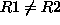or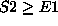or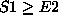. However each rule uses a different ``if...then'' statement. For example the first rule says that if R1=R2 and E1>S2 then.

In order to use constraint handling rules, it is necessary to translate them into the underlying ECLiPSe language using an automatic translator. The constraints must be written to a file called file.chr - in our example we shall use chrTaskResource.chr. To illustrate the loading and use of constraint handling rules, we give some example queries below.

```[eclipse 1]: lib(chr), lib(fd).

*       yes.

[eclipse 3]: chrTR(S1, R1, S2, R2), R1#=r1, R2#=r1.
*       S1 = S1{[0..100]}
*       S2 = S2{[0..100]}
*       R1 = r1
*       R2 = r1
*       yes.

[eclipse 4]: chrTR(S1, R1, S2, R2), R1=r1, R2=r1, S1#<=65.
*       S2 = S2{[50..100]}
*       R1 = r1
*       R2 = r1
*       S1 = S1{[0..50]}
*       yes.

[eclipse 5]: chrTR(S1,R1,S2,R2), R1=r1, S2#>=35, S2#<=45.
*       S1 = S1{[0..100]}
*       R2 = R2{[r2, r3]}
*       R1 = r1
*       S2 = S2{[35..45]}
*       yes.```

Query 3 yields less propagation than propiaTR because this implementation does not punch holes in the variables' domains.

Query 4 does, however, produce new information, because not only do both tasks use the same resource, but also the constraint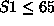means that taskmust precede task. The constraint deduces that the latest start time for S1 is actually 50, and the earliest start time for S2 is also (by coincidence) 50.

Query 5 uses the fact that the tasks must overlap to remove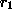from the domain of R2.

The chr library offers many more facilities, including multi-headed rules, and augmentation rules. These facilities can be explored in detail by studying the relevant chapter in [Be97], and trying out the example constraint handling rule programs which are distributed with ECLiPSe.Next: Explicit Data Driven Control Up: Complex Constraints Previous: The propia (Generalised Propagation)

Joachim Schimpf
Wed Sep 3 18:07:19 BST 1997